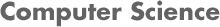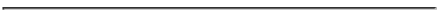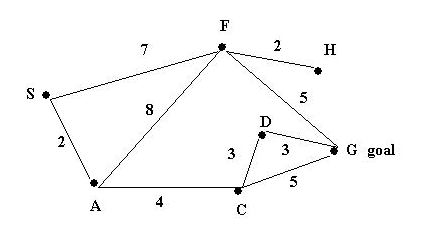### CS534 Artificial Intelligence Homework 1 - Fall 2007

#### PROF. CAROLINA RUIZ

Due Date: At the beginning of class on September 13, 2007.• Read Chapters 1, 2, 3, and 4 of the textbook. Chapters 3 and 4 should be read in more detail than Chapters 1 and 2.
• Read the online syllabus in detail.
• Go to Interesting AI Demos and Projects
• Browse around that website
• Choose a demo/project that you like on that website
• Study that demo/project in detail
• Look for related material online and in the textbook(s)
• Be ready to report on this AI demo/project during our next class.
• Look at some of the search demos posted on the textbook webpage.
• Problems: (you need to turn in written solutions to the problems below at the beginning of class on September 13, 2007)
1. Define Artificial Intelligence in your own words.
2. Provide two alternative definitions of AI, one focused on reasoning and the other one focused on acting intelligently.
3. What's the difference between the engineering and the scientific goals of AI? Explain your answer.
4. Suppose that you need to find a path between S and G in the following graph. The number attached to each edge in the graph represents the COST of traversing the edge.Assume also that the ESTIMATED distances to the goal are given by the following table:

 Node: S A C D F G H Estimated Distance to G: 10 5 4 3 4 0 2

For EACH of the following search methods:

• (10 points) Construct the search tree and mark in it the order in which the nodes/paths are EXPANDED by the search method while looking for a solution. When everything else is equal, order the children of a node in alphabetical order.
• (10 points) Display the state of the queue after each expansion of a node in the search tree.
• (3 points) Write down the solution path from S to G (if any) found by the method.
• (2 point) Write down the cost of the solution path found by the method.
As an illustration, the solution for Depth 1st search is provided below. For more illustrations, see Solutions to old HW1.

1. Depth 1st Search

Search Tree:

```          S
/ \
A   F
/ \
C   F
/ \
D   G
|
G
* goal
```

Queue:

```Depth First
Expanded  Queue
S      [(S)]
A      [(A,S) (F,S)]
C      [(C,A,S) (F,A,S) (F,S)]
D      [(D,C,A,S) (G,C,A,S) (F,A,S) (F,S)]
G      [(G,D,C,A,S) (G,C,A,S) (F,A,S) (F,S)]
goal reached!
```

List of expanded nodes: [S, A, C, D, G]

Resulting path: SACDG

Cost of resulting path: 12

2. (25 points) Breadth 1st Search

Search Tree:

Queue:

List of expanded nodes:

Resulting path:

Cost of resulting path:

3. (25 points) Iterative Deepending

Search Tree:

Queue:

List of expanded nodes:

Resulting path:

Cost of resulting path:

4. (25 points) Branch-and-Bound with Dynamic Programming (= Uniform Cost Search)

Search Tree:

Queue:

List of expanded nodes:

Resulting path:

Cost of resulting path:

5. (25 points) Greedy Search (= Best 1st Search)

Search Tree:

Queue:

List of expanded nodes:

Resulting path:

Cost of resulting path:

6. (25 points) A*

Search Tree:

Queue:

List of expanded nodes:

Resulting path:

Cost of resulting path:

7. (25 points) Hill-climbing

Search Tree:

Queue:

List of expanded nodes:

Resulting path:

Cost of resulting path:

8. (25 points) Beam search (w=2)

Search Tree:

Queue:

List of expanded nodes:

Resulting path:

Cost of resulting path: